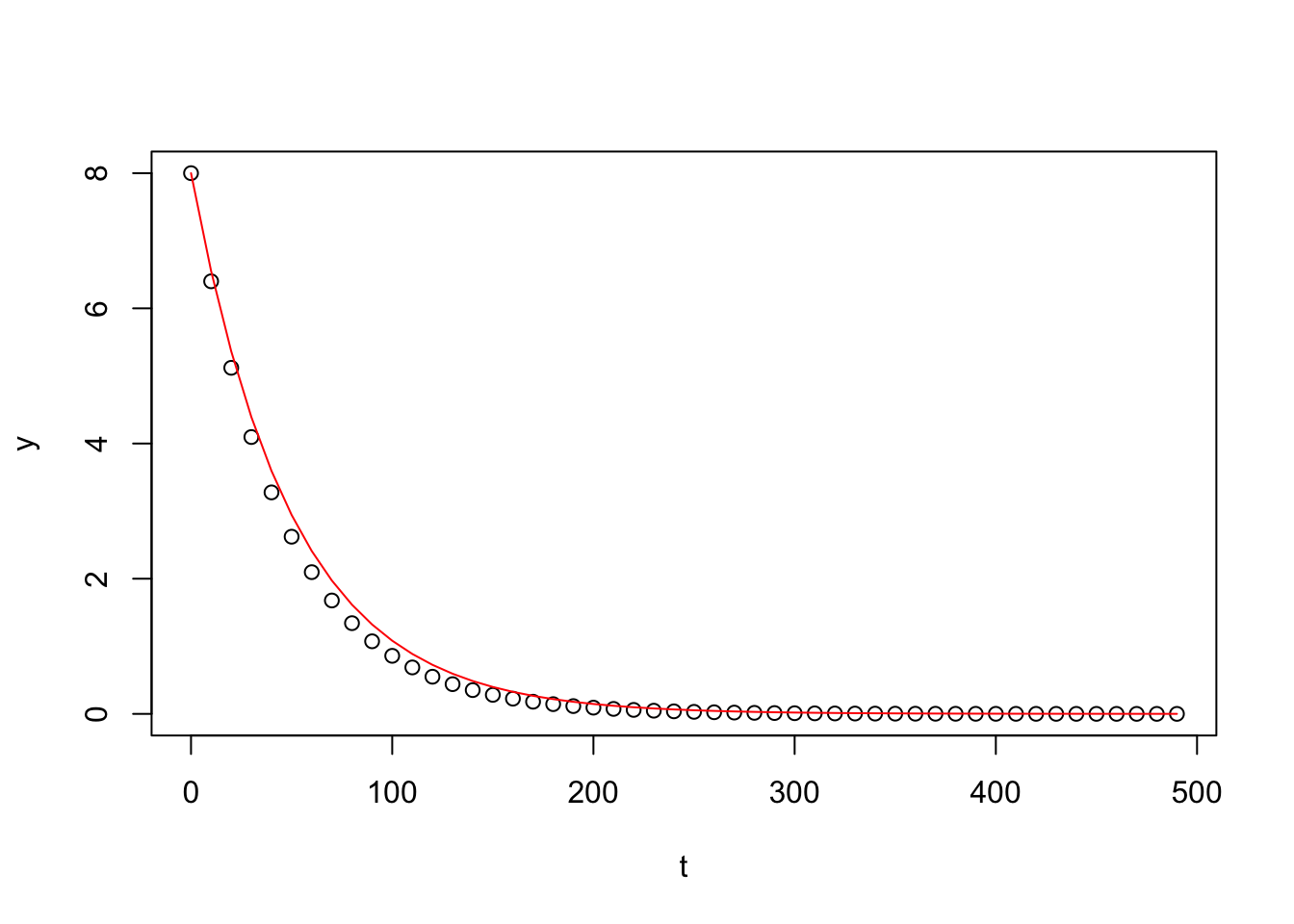### Prof. Dr. Gerrit Lohmann

#### klick here for the zoom session

Time required for each lecture: 7 h

### 9) Numerical Schemes incl. Random Systems

#### Material:

Gershenfeld Random

Gershenfeld finite elements

#### Learning outcome:

Examples of Resolution (global spectral model)

Ocean circulation models and boundary conditions

Classes of models:
• Ordinary diffential eq. (Box models)
• Partial diffential eq. (Diffusion & Advection)
• Stochastic (different time & length scales)

2D Staggered grids: Arakawa

Fourier–Galerkin: Spectral method

Finite differences and finite element methods

Example: Ocean model in finite elements

Numerics: Euler integrator

Leapfrog scheme

Coarse graining -> Stochastic equations; Climate Application

• ### Exercise I: Euler integrator

1. Play with the parameter h which is the step size in time units !

2. Can you find a crtical h when the numerical scheme does not work anymore?

3. Which value of h shall be taken in order to have an accurate solution (close to the analytical one) ?

#demonstration of Euler method in 1st order ODE: dy/dt=A*y

#the function dy/dt<-f(y,A,t)
f<-function(y,A,t)
{
return(A*y)
}

#constants
A<-  -0.02  #growth / decay rate
T<-  500    #integration time in time units
h<-  10     #step size in time units
Y0<- 8   #inital value

n<-T/h          #number of time steps (time / timestep)
t<-(0:(n-1))*h  #create a vector of discrete timesteps
y<-vector()     #define an empty vector for the state variable y(t)

#integration loop
for (i in 1:(n-1))
{
y[i+1]<-y[i]+h*f(y[i],A,t[i])   #Euler forward: y[t+h]<-y[t]+h*A*y[t]
}

plot(t,y,type="p") #plot the result against time

#additionaly plot the analytical solution in red
lines(t,Y0*exp(A*t),col="red",type="l")### Exercise II: Leapfrog scheme

studying Rossby and Kelvin waves in the shallow water equations.

### Literature:

• Gershenfeld, N. The nature of mathematical modeling, Cambridge University Press, Cambridge, 2003, 344 pp.

• Chirila, D., and G. Lohmann, 2015: Introduction to Modern Fortran for Earth System Sciences. 2015, XXII, 250 pages. 15 illus., 10 illus. in color. ISBN: 978-3-642-37008-3; Springer, Berlin Heidelberg. link, link to source code, link to source

• Goose, H., Climate system dynamics and modelling, Cambridge University Press, Cambridge, 2015, 358 pp.

• Press, W. H., S. A. Teukolsky, W. T. Vetterling, B. P. Flannery, “Numerical Recipes: The Art of Scientific Computing, 3rd Edition” Cambridge University Press, 2007, 1235 pp. http://www.nr.com/

• Karline Soetaert, Thomas Petzoldt and R. Woodrow Setzer, 2010. Solving Differential Equations in R. The R Journal Vol. 2/2, December 2010

• Cushman-Roisin, B. and Beckers, J.-M., Introduction to Geophysical Fluid Dynamics: Physical and Numerical Aspects

• Robert Cook et al., Concepts and Applications of Finite Element Analysis, John Wiley & Sons, 1989

• Harish, Ajay. (21 Mar 2019). Finite Element Method – FEM and FEA Explained. SimScale.

• Lohmann, G., 2020: Climate Dynamics: Concepts, Scaling and Multiple Equilibria. Lecture Notes 2020, Bremen, Germany. (pdf of the full script)

• R Core Team (2013). R: A language and environment for statistical computing. R Foundation for Statistical Computing, Vienna, Austria. An Introduction to R

• Paul Torfs, P., and & Claudia Brauer, C., 2014: A (very) short introduction to R